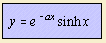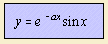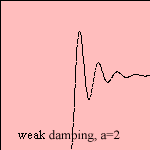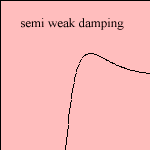# damped harmonic oscillation

## exponential

last updated: 2003-07-19

Suppose a harmonic oscillation that is damped by a force, which is magnitude proportional to the velocity 1). This is called a free damped harmonic oscillation. There are three possible situations, which are treated on this page.
The following curves give the amplitude as function of time.

## strong dampingThe function is the product of an exponential function and a hyperbolic sine.

## weak dampingThe function is the product of an exponential function and a sine.

## semi weak dampingThe function is the product of an exponential function and a line.

notes

1) Differential equation: y'' + a y' + b y = 0 with y(0) = 0.

Kuipers 1966 p.345.